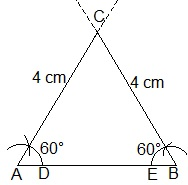Guru

# Construct an equilateral triangle, given its side and justify the construction. Q.5

• 0

what is the tricky way for solving the question of class 9th ncert math of Constructions chapter of ncert of exercise 11.1of math give me the best and simple way for solving this question in easy and simple way of question no.5 Construct an equilateral triangle, given its side and justify the construction.

Share

1. Construction Procedure:

1. Let draw a line segment AB = 4 cm .

2. With A and B as centres, draw two arcs on the line segment AB and note the point as D and E.

3. With D and E as centres, draw the arcs that cuts the previous arc respectively that forms an angle of 60° each.

4. Now, draw the lines from A and B that are extended to meet each other at the point C.

5. Therefore, ABC is the required triangle.Justification:

From construction, it is observed that

AB = 4 cm, ∠A = 60° and ∠B = 60°

We know that, the sum of the interior angles of a triangle is equal to 180°

∠A+∠B+∠C = 180°

Substitute the values

⇒ 60°+60°+∠C = 180°

⇒ 120°+∠C = 180°

⇒∠C = 60°

While measuring the sides, we get

BC = CA = 4 cm (Sides opposite to equal angles are equal)

AB = BC = CA = 4 cm

∠A = ∠B = ∠C = 60°

Hence, justified.

• 0